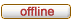# Horde3D

Next-Generation Graphics Engine
 It is currently 08.12.2021, 10:45

 All times are UTC + 1 hour

 Print view Previous topic | Next topic
Author Message
 Post subject: Animation Layer remaningWeight computationPosted: 23.11.2011, 10:51Joined: 10.02.2011, 07:53
Posts: 2
Hello,
I found a Bug in the animation system, more precisely in the computation for the remainingWeight for animation layers

Now, the Horde3D goes through all stages, stating at the highest layer and going down to the lowest. While doing this the remainingWeight gets computed.
The way I see it, the remainingWeight should work like this: The highest Layer has priority so it takes as much weight as it needs from the remainingWeight Bucket. The second highest layer check if there is still something left and if so, has its pick. And so on.

If I understood this correctly, the remainingWeight should start at 1.0 and should steadily empty till reaching 0.
The thing is that this is not case with the current implementation (file egAnimation.cpp):

The Bug

Code:
remainingWeight *= 1.0f - minf( layerWeightSum, 1.0f );
//layerWeightSum = total weight of previous layer

This looks like the remainingWeight is indented to be used as a weight for layers weights. However, even if this is the case, there is a bug with the computation of the final weight:

Code:
weight = (curStage.weight / layerWeightSum) * remainingWeight;
//curStage.weight = weight of current stage
/layerWeightSum = total weight of current Layer

This calculation causes that each layer will render with the whole remaining weight, no matter how much weight it actually needs.
This means that the remainingWeight isn't used as a weight of the layer.

Example
Code:
Layer 3
remainingWeight = 1.0 * (1.0 - 0.0) = 1.0
curStage.weight = layerWeightSum = 0.5
-------------------------------------------------
render weight = 1 * remainingWeight = 1.0

Layer 2
remainingWeight = 1.0 * (1.0 - 0.5) = 0.5
curStage.weight = layerWeightSum = 0.6
-------------------------------------------------
render weight = 1 * remainingWeight = 0.5

Layer 1
remainingWeight = 0.5 * (1.0 - 0.6) = 0.2
curStage.weight = layerWeightSum = 0.3
-------------------------------------------------
render weight = 1 * remainingWeight = 0.2

Solution 1

To fix this bug we must alter the render weight computation:
Code:
weight = curStage.weight * (curStage.weight / layerWeightSum) * remainingWeight;

Now the System uses the remainingWeight as a weight for each layer.

Example:
Code:
Layer 3
remainingWeight = 1.0 * (1.0 - 0.0) = 1.0
curStage.weight = layerWeightSum = 0.5
-------------------------------------------------
render weight = 0.5 * 1 * remainingWeight = 0.5

Layer 2
remainingWeight = 1.0 * (1.0 - 0.5) = 0.5
curStage.weight = layerWeightSum = 0.6
-------------------------------------------------
render weight = 0.6 * 1 * remainingWeight = 0.3

Layer 1
remainingWeight = 0.5 * (1.0 - 0.6) = 0.2
curStage.weight = layerWeightSum = 0.3
-------------------------------------------------
render weight = 0.3 * 1 * remainingWeight = 0.06

Solution 2

However, I still find this wrong because it doesn't implement the remaining weight behavior as one would assume. Much better would be to implement the remainingWeight as some sorts of bucket from which the layers can take out weight in order of their priority (ids)
To achieve this I would change the calculations for the remainingWeight and weight:

Code:
remainingWeight = maxf(remainingWeight - minf( layerWeightSum, 1.0f ), 0.0f);

Code:
//render weight
if(layerWeightSum >= remainingWeight) //this layer will use all of the remaining weight
weight = (curStage.weight / layerWeightSum) * remainingWeight;
else //weight will still be available for lower layer
weight = curStage.weight;

And here is the example now with the proposed changes:
Code:
Layer 3
remainingWeight = 1.0 - 0.0 = 1.0
curStage.weight = layerWeightSum = 0.5
-------------------------------------------------
render weight = 0.5

Layer 2
remainingWeight = 1.0 - 0.5 = 0.5
curStage.weight = layerWeightSum = 0.6
-------------------------------------------------
render weight = 1 * remainingWeight = 0.5

Layer 1
remainingWeight = maxf(0.5 - 0.6) = 0
curStage.weight = layerWeightSum = 0.3
-------------------------------------------------
render weight = 1 * remainingWeight = 0

TopDisplay posts from previous: All posts1 day7 days2 weeks1 month3 months6 months1 year Sort by AuthorPost timeSubject AscendingDescending

 All times are UTC + 1 hour

#### Who is online

Users browsing this forum: No registered users and 1 guest

 You cannot post new topics in this forumYou cannot reply to topics in this forumYou cannot edit your posts in this forumYou cannot delete your posts in this forumYou cannot post attachments in this forum

Search for:
 Jump to:  Select a forum ------------------ Horde3D Usage    General Discussion    Support    Bug Reports    Asset Creation    Showcase    Advanced Rendering Techniques    Tools    Free Discussion Horde3D Development    Developer Discussion